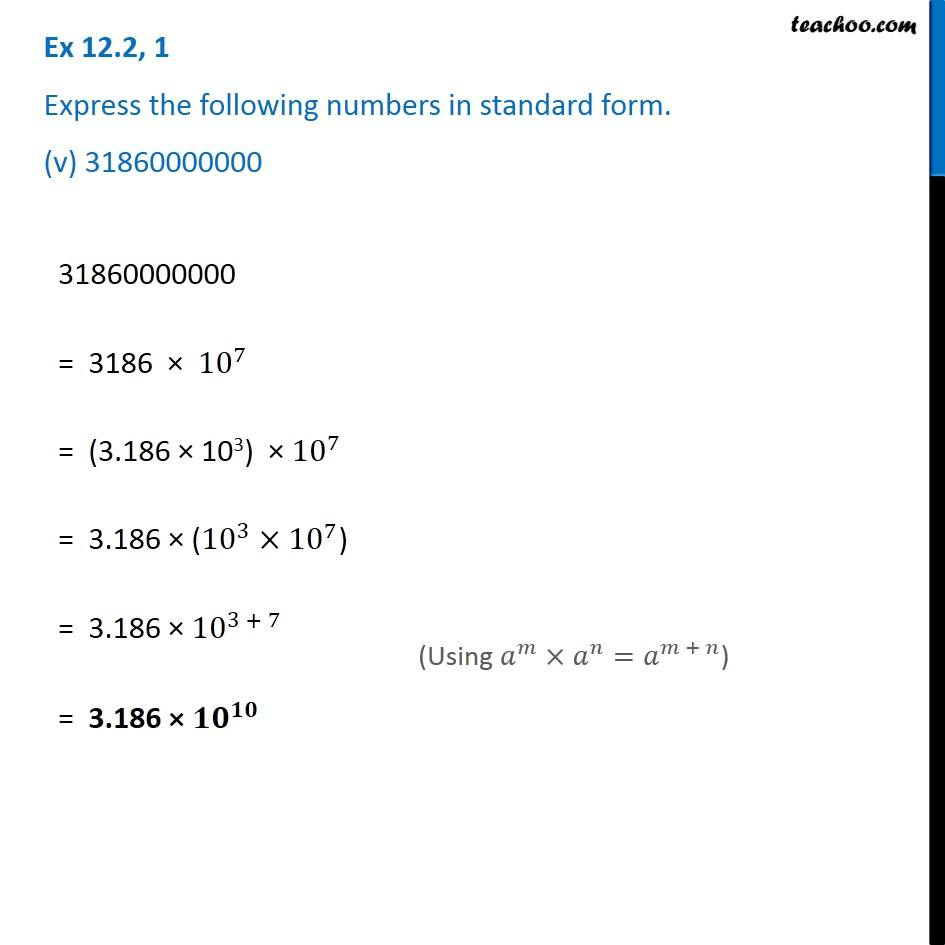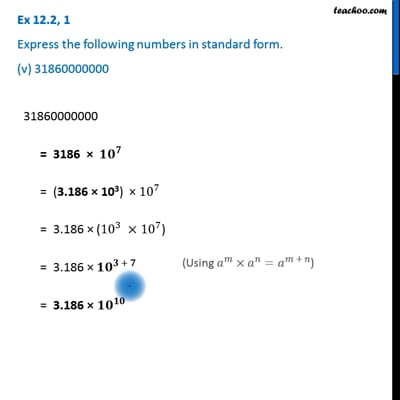Ex 12.2

Chapter 12 Class 8 Exponents and Powers
Serial order wiseThis video is only available for Teachoo black users

Get live Maths 1-on-1 Classs - Class 6 to 12

### Transcript

Ex 12.2, 1 Express the following numbers in standard form. (v) 31860000000 31860000000 = 3186 × 〖10〗^7 = (3.186 × 103) × 〖10〗^7 = 3.186 × (〖10〗^3×〖10〗^7) = 3.186 × 〖10〗^(3 + 7) = 3.186 × 〖𝟏𝟎〗^𝟏𝟎 (Using 𝑎^𝑚×𝑎^𝑛=𝑎^(𝑚 + 𝑛))